Select Page

Definition

The mode is defined as the value in the data, which occur the greatest number of times in an array of data.

### For example

i. The mode of the values 2, 5, 7, 8, 9, 9 and 10 is 9

ii. The mode of the values 11, 12, 12, 14, 15, 15, 15, 17, 17 and 19 is 15.

However, if each value occurs the same number of times then there will be no mode. There may be a case where two or more values occur the same number of times but more frequently than any other values, in such case there is more than one mode. In this perspective mode differs from median and mean because there is always single mean or median. In a distribution where there is only one mode is called uni-modal distribution. Whereas, a distribution having two modes is called bi-modal distribution and where there are more than two modes, such distribution shall be referred as multi-model distribution.

## Mode for Grouped Data

In a frequency distribution, having equal class interval sizes, the class with the highest frequency is called the modal class. The formula for calculating mode for grouped data, arranged in the form of frequency distribution, is provided as follows;

Where,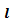= lower class boundary of the model class i.e. the class with the highest frequency.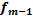= frequency of the class preceding the modal class.= frequency of the class following the modal class.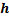= class interval size of the modal class.

### For example

The mode for the frequency distribution of weights of 120 students in a university will be calculated from Table 16, as follows;

Table 16

 Weight (lb) Frequency (f) Class Boundaries 110 – 119 1 109.5-119.5 120 – 129 4 119.5-129.5 130 – 139 17 129.5-139.5 140 – 149 28 139.5-149.5 150 – 159 25 149.5-159.5 160 – 169 18 159.5-169.5 170 – 179 13 169.5-179.5 180 – 189 6 179.5-189.5 190 – 199 5 189.5-199.5 200 – 209 2 195.5-209.5 210 -219 1 209.5-219.5

From the above table we have extracted the following;

By using the formula,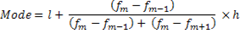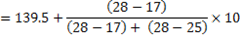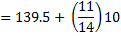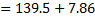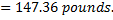## Mode for Discrete Data

In case of discrete data, the mode may be found at a glance since it is the most common value i.e. the value with greatest frequency.

### For example

The following table 17 gives the number of times various black cards appeared in 36 deals of 5 playing cards. The data are discrete because we may get 4 or 5 black cards but never 4.5 or 4.33 black cards. The most common number of black cards is 2, corresponding to the greatest frequency which is the mode.

Table 17

 Number of Black Cards Frequency 0 2 1 7 2 12 3 9 4 5 5 1
Shares